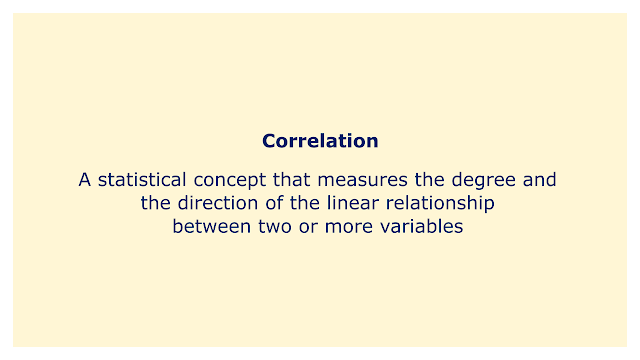# CorrelationImage: Moneybestpal.com

### A statistical concept known as correlation assesses the strength and direction of the linear link existing between two or more variables. Correlation shows the strength of the relationship between the variables and whether they are positively or negatively correlated. Correlation does not imply causation, which means that it just shows that two variables tend to move in tandem rather than that one causes or influences the other.

The intensity and direction of the link can be measured using various correlation coefficients, which are numerical values that range from -1 to +1. The most prevalent correlation coefficient is the Pearson correlation coefficient, which assesses the linear relationship between two continuous variables with a normal distribution. By dividing the covariance of the two variables by the sum of their standard deviations, the Pearson correlation coefficient is determined. In the event of a perfect positive linear correlation, a perfect negative linear correlation, and the absence of any linear correlation, the Pearson correlation coefficient is +1, -1, and 0 respectively.

Graphs known as scatterplots, which show the values of two variables as points on a Cartesian plane, can be used to visually express correlation. In addition to displaying the regression line or best-fit line, which illustrates the linear relationship between the variables, scatterplots can also depict the pattern, direction, and dispersion of the data points. Outliers, or data points that differ markedly from the overall trend of the data, can also be seen on scatterplots and may have an impact on the regression analysis and correlation coefficient.

The use of correlation in data analysis is crucial because it allows for the exploration of correlations between variables, the testing of hypotheses, the identification of prospective predictors, the management of confounding variables, and the evaluation of the reliability and validity of the data. To investigate the relationships between various phenomena, variables, and results, correlation is also frequently utilized in a wide range of disciplines and fields, including biology, economics, finance, psychology, and sociology.

There are some restrictions and difficulties associated with correlation, including the linearity presumption, the susceptibility to outliers, the potential for misleading correlations, the multicollinearity issue, and the challenge of determining causality. The Kendall correlation coefficient measures the concordance or discrepancy between two pairs of rankings, the partial correlation coefficient measures the correlation between two variables after controlling for the effect of one or more independent variables, and the Spearman correlation coefficient measures the rank correlation between two ordinal variables or two variables that have a monotonic relationship.
Tags